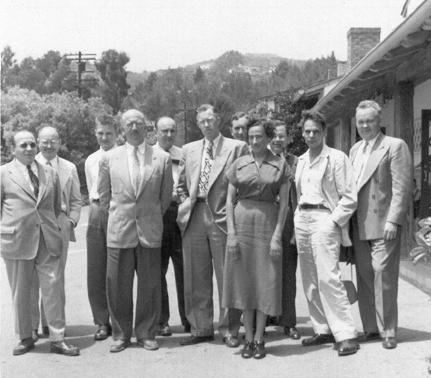# The Rosser Matrix

The Rosser matrix is a classic matrix eigenvalue test problem.

### Contents

#### The Rosser function

You may not have noticed the rosser function, although it has been on your path for as long as you have had MATLAB.

  which rosser

C:\Program Files\MATLAB\R2014a\toolbox\matlab\elmat\rosser.m


Here is the Rosser matrix.

  R = rosser

R =

611   196  -192   407    -8   -52   -49    29
196   899   113  -192   -71   -43    -8   -44
-192   113   899   196    61    49     8    52
407  -192   196   611     8    44    59   -23
-8   -71    61     8   411  -599   208   208
-52   -43    49    44  -599   411   208   208
-49    -8     8    59   208   208    99  -911
29   -44    52   -23   208   208  -911    99



Here is most of the help entry.

rosser Classic symmetric eigenvalue test problem.
This matrix was a challenge for many matrix eigenvalue algorithms.
But LAPACK's DSYEV routine used in MATLAB has no trouble with it.
The matrix is 8-by-8 with integer elements.
It has:
* A double eigenvalue.
* Three nearly equal eigenvalues.
* Dominant eigenvalues of opposite sign.
* A zero eigenvalue.
* A small, nonzero eigenvalue.

#### The eigenvalues

Here are the eigenvalues.

  format long
e = eig(R)

e =

1.0e+03 *

-1.020049018429998
0.000000000000000
0.000098048640722
1.000000000000000
1.000000000000000
1.019901951359278
1.020000000000000
1.020049018429997



As the help entry says, there is a double eigenvalue at 1000; there are three nearly equal eigenvalues around 1020; the largest positive eigenvalue is matched in magnitude by the largest negative eigenvalue; there is an exact zero eigenvalue; and there is one relatively small eigenvalue near .098.

When I was a student fifty years ago just learning about matrix computation, there were several competing methods for matrix eigenvalue computation. Francis and Wilkinson were just publishing their work on the QR algorithm, and EISPACK and MATLAB were years in the future. So the Rosser matrix represented a serious test of the methods we had available at the time. I have always admired the fact that Rosser had constructed one elegant matrix whose eigenvalue problem contained all of these challenges.

Today, the symmetric QR algorithm with Wilkinson's shift can easily handle the Rosser matrix. But we still like to have it in our collection to use in testing other algorithms.

#### Exact solution

Using the Symbolic Toolbox, we can compute the exact eigenvalues. First, find the characteristic polynomial.

  p = poly2sym(charpoly(R),'x')


p =

x^8 - 4040*x^7 + 5080000*x^6 + 82518000*x^5 - 5327676250000*x^4 + 4287904631000000*x^3 - 1082852512000000000*x^2 + 106131000000000000*x



The matrix is singular, so the constant term is zero. The polynomial has degree eight, but it factors nicely into linear and quadratic factors.

  f = factor(p)


f =

x*(x - 1020)*(x^2 - 1040500)*(x^2 - 1020*x + 100)*(x - 1000)^2



These low degree factors make it possible to obtain exact symbolic expressions for the eigenvalues.

  e = solve(f)


e =

0
1000
1020
1000
10*10405^(1/2)
-10*10405^(1/2)
100*26^(1/2) + 510
510 - 100*26^(1/2)



However, these symbolic expressions do not immediately reveal the numerical properties that interest us.

#### J. Barkley Rosser

J. Barkley Rosser, Sr., was a colleague of my mentors George Forsythe and John Todd at the Institute for Numerical Analysis at UCLA in the 1950s. He is the most prominent figure in the front row of this photo of the INA members that I use near the beginning of my talk about "The Evolution of MATLAB". Forsythe is in the center of the group and Todd is in the back row, looking over Forsythe's shoulder.Rosser spent much of his career as director of the famous Army Mathematics Research Center at the University of Wisconsin in Madison. He is best known for his work in number theory and logic. In 1936, he developed a version of Godel's incompleteness theorem that is now referred to as Rosser's trick. Stated informally, it says "For every proof of a theorem, there is a shorter proof of its negation.".

Published with MATLAB® R2014a

|

### コメント

コメントを残すには、ここ をクリックして MathWorks アカウントにサインインするか新しい MathWorks アカウントを作成します。